[Tech +]Create a point cloud using a depth map. How? Like this!

Hello, my name is Layton, and I research and develop the core technologies in Arbeon’s AR team.

This is the second article following what was covered by the AR team on
3D images (read article). Let’s start right away.

Creating a point cloud

based on the pinhole camera model

We have explained on what a depth map is among the types of 3D images in the previous article. By utilizing the created depth map, it is generally possible to create a 3D point cloud. In this article, we will talk about the mechanism of how depths can be measured using the pinhole camera model and how we can use it to create a point cloud.

Expressing depth map using a pinhole camera model

In general, the depths of pixels in depth maps measured by depth cameras such as LiDAR show how far each specific location is from the camera.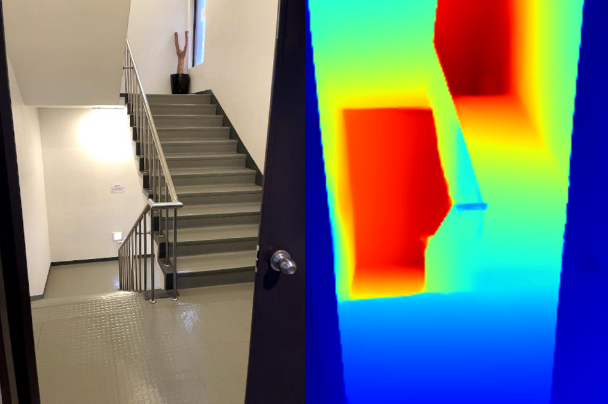The image above is the result of taking a depth image that corresponds to the color image of the emergency stairs using the LiDAR on the iPhone 13 Pro. Here, the regions close to the camera are shown as blue, while the far regions are shown as red. You can notice that the door and hallway close to the camera are shown as blue, while the wall near the hallway, which is far away from the camera, is shown as red.

The actual mechanism of taking these color images and depth maps is quite complex, as its structure includes passing by multiple layers of lenses. However, for us to calculate the coordinates of a point cloud, we shall assume that the simplified model of pinhole cameras was used to take the image. A pinhole camera has a small pin-shaped hole on a rectangular box, wherein the light that enters through the hole hits the opposite side of the surface and forms an image. It is the most basic model that can express the mechanism of taking a picture.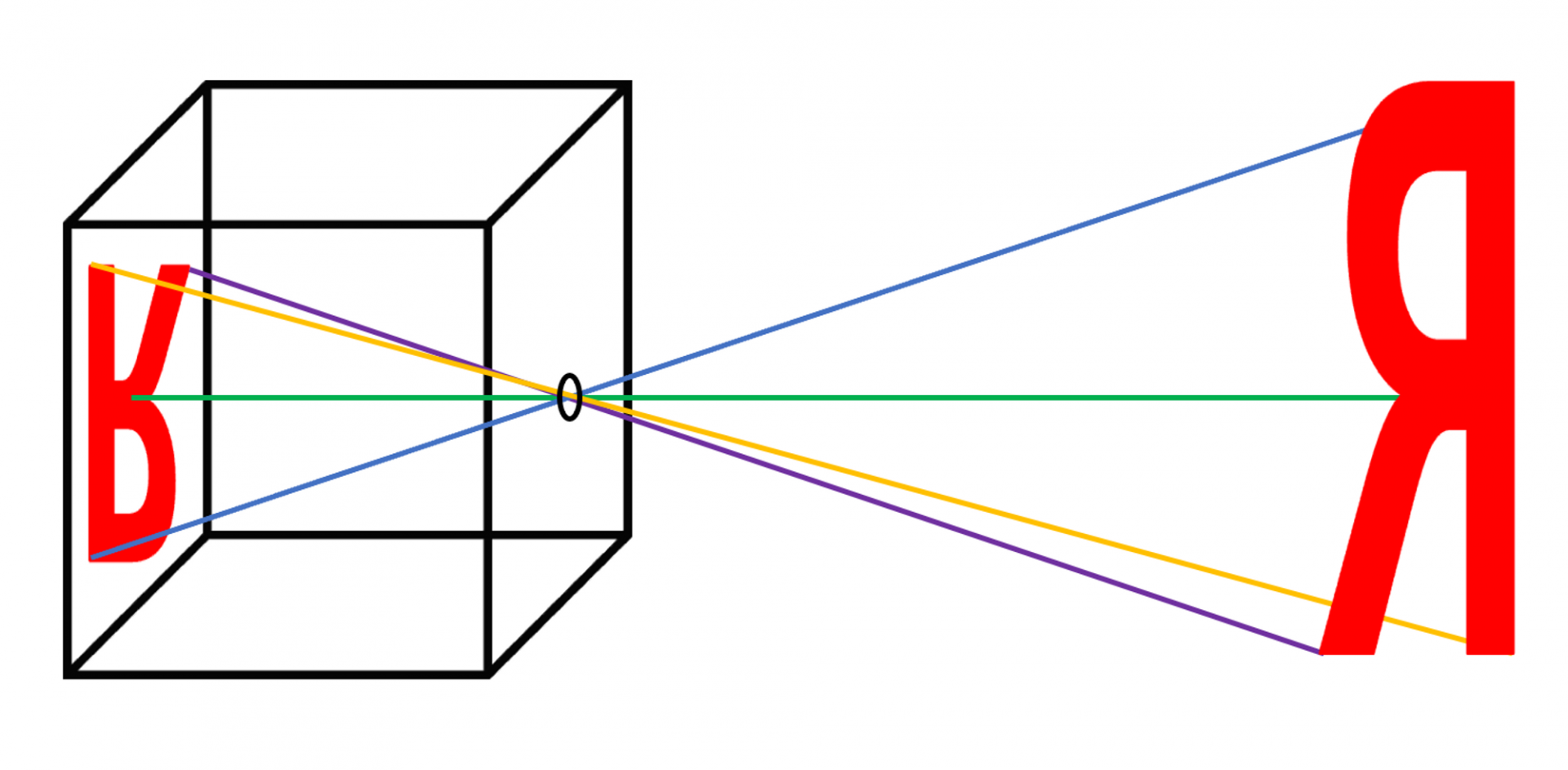An example of a pinhole camera model

Given a real-world object R, as shown in the above figure, the real-world light is reflected as it hits R, and the reflected light goes into the camera through the pinhole. It then forms an inverted and reversed image of the object. Due to this mechanism, images obtained from cameras are always reversed at some point. Therefore, it involves an additional operation of converting the images back to its original form.

Creating a point cloud with camera’s internal parameters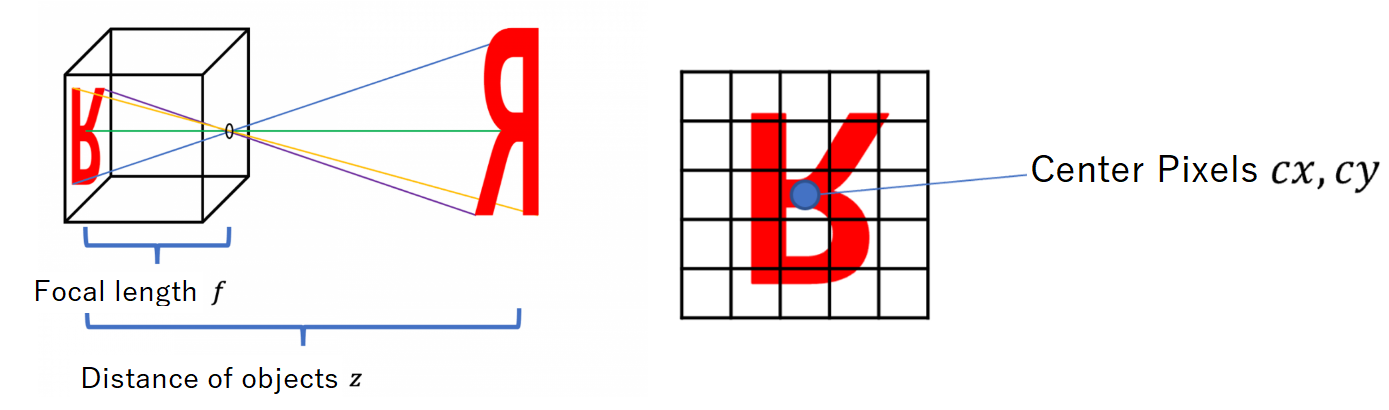Each pixel value of the depth map expressed by the pinhole camera model is determined by several important parameters of the camera. Inversely, by utilizing the parameters that determine the distance, we can estimate the actual location of the objects in the depth map and express it as a point cloud.

First, here are some important values that are required to calculate the coordinates of the point cloud.

1. Optical center: In the depth camera, the pixel that corresponds to the central point of the point symmetry (i.e, the center of the pinhole) is called the optical center. The x- and y- axis of the optical center are generally expressed as cx and cy. Their units are pixels.
2. Focal length: The distance from the camera’s pinhole to the sensor where the image is formed is called the focal length. Its unit is pixel too. They are classified as fx and fy in case the sensor’s vertical and horizontal lengths are different. (Although it has a different meaning from the focal length in optical science, this concept is used in image processing for convenience.)
3. Depth: The vertical distance from the actual object to where the image is formed is defined as depth. It is expressed as z, and its unit is meter.

If you have followed up to this point, you will be able to create a point cloud using the given data.
If you are aware of the focal length, depth, and optical center of the camera, you can calculate the actual coordinates of each depth’s point cloud using the formula below.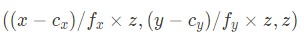I will explain its principle in detail through a figure.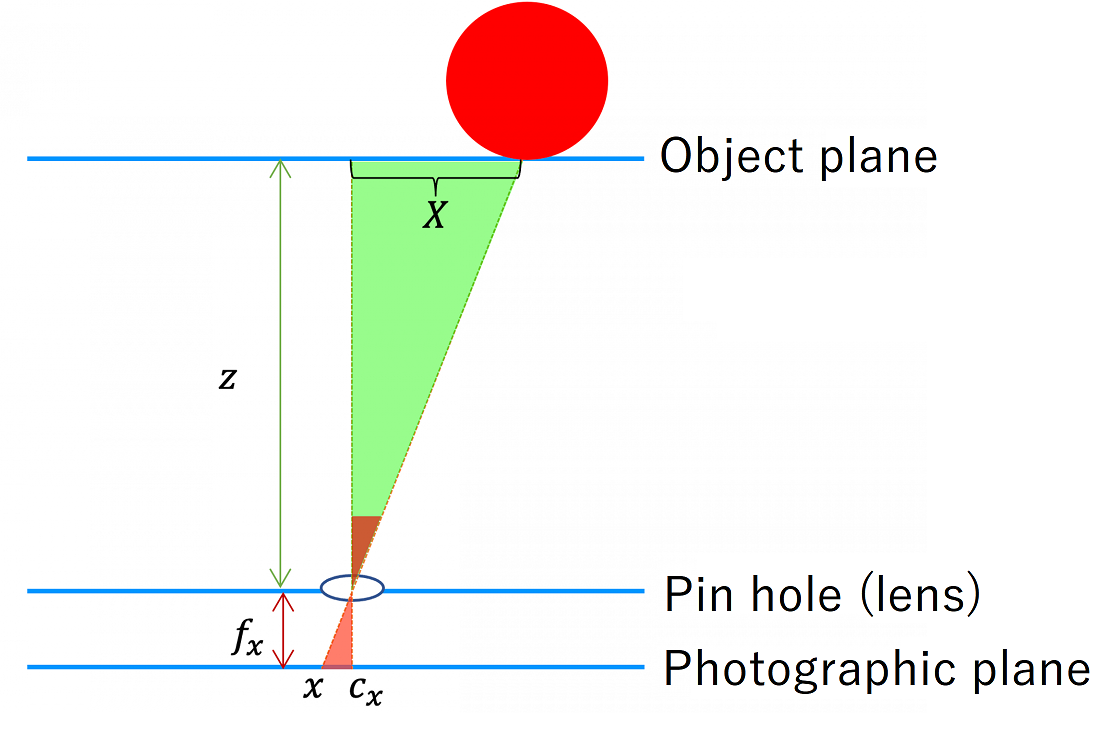Let's assume that the center of the red ball is formed at pixel x in the picture, as shown in the above figure. Then, pixel x, optical center cx, and the pinhole form a red right-angled triangle. However, if the position is set to 0 where the optical center is located at a distance of z, and the distance to the red ball is X, then the following equation can be formed according to the triangle’s proportional relationship: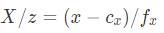Therefore, the following can be obtained by multiplying z to both sides: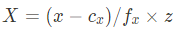With the same principle, let's assume the distance based on the y-axis is called Y. Then the following equation can be obtained: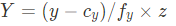Finally, for the Z-coordinate, use the depth information of z as it is.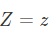If you group the calculated x,y, and z coordinates in a bracket, the following equation is obtained: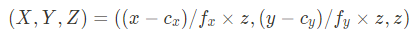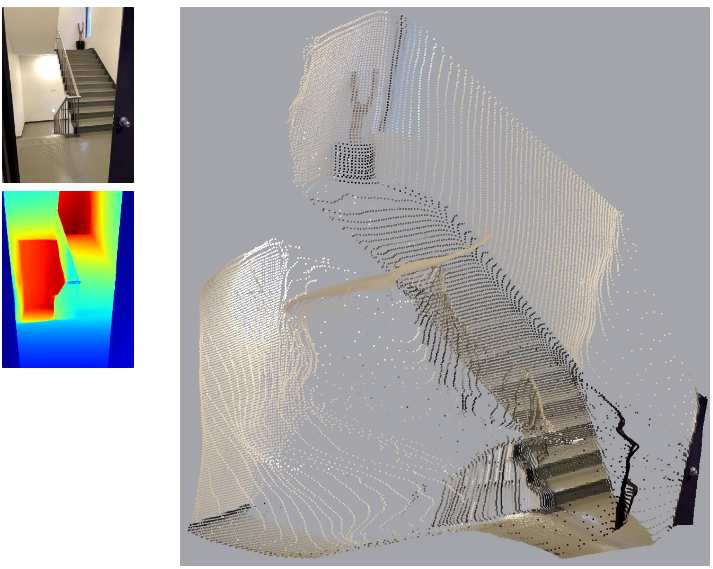The above figure is the result of creating the point cloud using the depth map and color image of the stairs.
You can check that the hallways upstairs and downstairs are expressed well, and the floor and walls are also distinguished vertically.

However, the surface is not as perfectly flat as expected, and there are some small distortions for the locations of handrails or stairs. Typically, these distortions happen due to the differences in the actual depth and measured depth, as there are noises in the process of taking a depth map.

These noises may occur due to the limitation in the camera's performance or the error caused by the diffused reflection of the laser on transparent or shiny materials, etc. Recently, the research on restoring the errors in depth information through deep learning has been quite active.

Summary

We went through how to create a point cloud based on the pinhole camera model. We have covered the mechanism of the pinhole cameral in taking images and the formula to convert the depth map into the coordinates of a 3D point cloud using the focal length, optical center, and depth information. Based on these mechanisms, we created a point cloud using an actual depth map taken with an iPhone, and we were able to confirm that the point cloud expresses the 3D shape of the real-world object effectively.

If we apply this technique one step further, we can consider the method of taking images of one object from several locations using the depth camera, converting these into point cloud, and combining them. In this case, not only the internal parameters of the camera, but also extrinsic camera parameters, which express the camera’s location and position, are required.

In the next article, we will cover how to find these extrinsic camera parameters, so please stay tuned.

Thank you for reading this long article.

:)

Ref.

https://ko.wikipedia.org/wiki/

https://medium.com/yodayoda/from-depth-map-to-point-cloud-7473721d3f

https://www.apple.com/am/iphone-13-pro/specs/